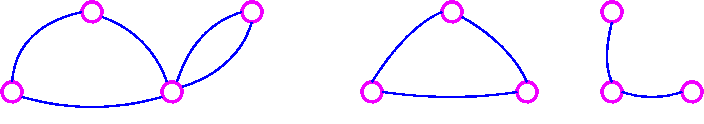# 6.1: Graph Theory

•• Maxie Inigo, Jennifer Jameson, Kathryn Kozak, Maya Lanzetta, & Kim Sonier
• Coconino Community College
$$\newcommand{\vecs}{\overset { \rightharpoonup} {\mathbf{#1}} }$$ $$\newcommand{\vecd}{\overset{-\!-\!\rightharpoonup}{\vphantom{a}\smash {#1}}}$$$$\newcommand{\id}{\mathrm{id}}$$ $$\newcommand{\Span}{\mathrm{span}}$$ $$\newcommand{\kernel}{\mathrm{null}\,}$$ $$\newcommand{\range}{\mathrm{range}\,}$$ $$\newcommand{\RealPart}{\mathrm{Re}}$$ $$\newcommand{\ImaginaryPart}{\mathrm{Im}}$$ $$\newcommand{\Argument}{\mathrm{Arg}}$$ $$\newcommand{\norm}{\| #1 \|}$$ $$\newcommand{\inner}{\langle #1, #2 \rangle}$$ $$\newcommand{\Span}{\mathrm{span}}$$ $$\newcommand{\id}{\mathrm{id}}$$ $$\newcommand{\Span}{\mathrm{span}}$$ $$\newcommand{\kernel}{\mathrm{null}\,}$$ $$\newcommand{\range}{\mathrm{range}\,}$$ $$\newcommand{\RealPart}{\mathrm{Re}}$$ $$\newcommand{\ImaginaryPart}{\mathrm{Im}}$$ $$\newcommand{\Argument}{\mathrm{Arg}}$$ $$\newcommand{\norm}{\| #1 \|}$$ $$\newcommand{\inner}{\langle #1, #2 \rangle}$$ $$\newcommand{\Span}{\mathrm{span}}$$$$\newcommand{\AA}{\unicode[.8,0]{x212B}}$$

There are several definitions that are important to understand before delving into Graph Theory. They are:

• A graph is a picture of dots called vertices and lines called edges.
• An edge that starts and ends at the same vertex is called a loop.
• If there are two or more edges directly connecting the same two vertices, then these edges are called multiple edges.
• If there is a way to get from one vertex of a graph to all the other vertices of the graph, then the graph is connected.
• If there is even one vertex of a graph that cannot be reached from every other vertex, then the graph is disconnected.

## Example $$\PageIndex{1}$$: Graph ExampleFigure $$\PageIndex{1}$$: Graph 1

In the above graph, the vertices are U, V, W, and Z and the edges are UV, VV, VW, UW, WZ1, and WZ2.

This is a connected graph. VV is a loop. WZ1, and WZ2 are multiple edges.

## Example $$\PageIndex{2}$$: Graph ExampleFigure $$\PageIndex{2}$$: Graph 2 Figure $$\PageIndex{3}$$: Graph 3

The graph in Figure $$\PageIndex{2}$$ is connected while the graph in Figure $$\PageIndex{3}$$ is disconnected.

## Definition: Graph Concepts and Terminology

• Order of a Network: the number of vertices in the entire network or graph
• Adjacent Vertices: two vertices that are connected by an edge
• Adjacent Edges: two edges that share a common vertex
• Degree of a Vertex: the number of edges at that vertex
• Path: a sequence of vertices with each vertex adjacent to the next one that starts and ends at different vertices and travels over any edge only once
• Circuit: a path that starts and ends at the same vertex
• Bridge: an edge such that if it were removed from a connected graph, the graph would become disconnected

## Example $$\PageIndex{3}$$: Graph TerminologyFigure $$\PageIndex{4}$$: Graph 4

In the above graph the following is true:

• Vertex A is adjacent to vertex B, vertex C, vertex D, and vertex E.
• Vertex F is adjacent to vertex C, and vertex D.
• Edge DF is adjacent to edge BD, edge AD, edge CF, and edge DE.

The degrees of the vertices:

 A 4 B 4 C 4 D 4 E 4 F 2

Here are some paths in the above graph: (there are many more than listed)

A,B,D

A,B,C,E

F,D,E,B,C

Here are some circuits in the above graph: (there are many more than listed)

B,A,D,B

B,C,F,D,B

F,C, E, D, F

The above graph does not have any bridges.

This page titled 6.1: Graph Theory is shared under a CC BY-SA 4.0 license and was authored, remixed, and/or curated by Maxie Inigo, Jennifer Jameson, Kathryn Kozak, Maya Lanzetta, & Kim Sonier via source content that was edited to the style and standards of the LibreTexts platform; a detailed edit history is available upon request.